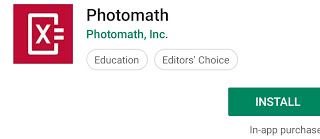•## மாணவர்களுக்கு உதவும் Easy Camera CalculatorKEY FEATURES
Scan textbook (print) AND handwritten problems
Scientific calculator
Step-by-step explanations for every solution
Multiple solving methods
No internet connection required to use
30+ languages supported
Interactive graphs
MATH TOPICS
Basic Math/Pre-Algebra: arithmetic, integers, fractions, decimal numbers, powers, roots, factors
Algebra: linear equations/inequalities, quadratic equations, systems of equations, logarithms, functions, matrices, graphing, polynomials
Trigonometry/Precalculus: identities, conic sections, vectors, matrices, complex numbers, sequences and series, logarithmic functions
Calculus: limits, derivatives, integrals, curve sketching Statistics: combinations, factorials
Our in-house team of veteran math educators also partner with teachers worldwide to ensure we’re utilizing the most effective, contemporary teacher methodologies in our math engines.
Featured in Huffington Post, Forbes, TIME, CNN, EdSurge, Guiding Tech, The Verge, TechCrunch and more.
Photomath is and will always be free to use, but you can boost your learning by upgrading to Photomath Plus. Photomath Plus provides solutions to full textbooks and problem sets with word problems! Currently restricted to the US and specific textbooks only.